# Creating a calculation field

A calculation field defines a mathematical expression, which can be a final calculation or an intermediate value to be used in another calculation. See below for how to create a calculation field. This topic also includes information on the following:

 1 Select Design > Fields to create.

You can select the Fields to create function from any of the Design function windows, enabling you to create temporary fields while specifying other report information.

When you select Fields to create, the Temporary Fields list is displayed. If you are creating a new report or adding temporary fields for the first time, the Available Field Types list is also displayed.

 2 From the Available Field Types list, select Calculation Fields.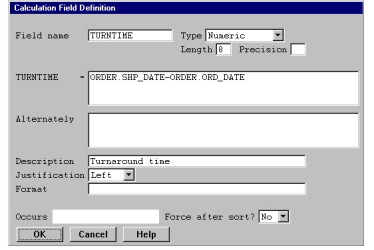3 In the Calculation Field Definition window, enter data in each field as instructed below.

As an example, we’ve defined a calculation field that contains the turnaround time between the day an order is taken (ORD_DATE) and the day the product is shipped (SHP_DATE).

Field name

Enter a name to identify the field. This name must be unique among all temporary fields. It must begin with a letter and the remaining characters can be letters, digits, underscores (_), or dollar signs (\$).

We called our field TURNTIME.

Type

From the displayed selection window, select the data type of the calculation’s result:

Alphanumeric

Numeric

Date

Time

You can use alphanumeric calculation fields to assign a string into a field (the calculation field) based on some condition. This calculation field can then be printed and will contain one of two strings based on some set of criteria.

If the result of the calculation is to be a date or time value, keep in mind that only limited calculations can be performed on dates and times. See the table below for the types of calculations that can be performed and the result type. See Performing calculations on date and time fields for more information about date and time calculations.

Calculation

Result type

date field +/– date field = # of days

Numeric

time field +/– time field = # of minutes

Numeric

(date field + time field) – (date field + time field) = # of days – HHMM

Time

date field +/– numeric field(s) or literal(s) = YYMMDD

Date

time field +/– numeric field(s) or literal(s) = # of days – HHMM

Time

Since TURNTIME will contain the difference between two dates, the data type must be Numeric.

Length

Enter the desired length for the calculation’s result.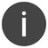When using date or time fields in your calculation, the length should match the storage length of the base value in the expression. For example, in the fourth rule above, the length of the calculation field should be the same as the length of the date field.

In our example, the length of the field is 8.

Precision

If the result is to be an implied-decimal value, enter the number of digits to the right of the decimal point. The maximum precision is 10. If none of the operands in the calculation expression are implied-decimal, you must multiply by 1.0 to get an implied-decimal result.

Our example field is not implied-decimal, so we left this field blank.

FIELD NAME =

FIELD NAME is the name of the field you entered in the Field name field above. (For example, in figure 1, the prompt is TURNTIME = .) Enter the calculation(s) you want ReportWriter to perform when it encounters this field while generating a report.

Your expression can be composed of

• operands (literals and field names).
• arithmetic operators (+ for addition, for subtraction, * for multiplication, and / for division).
• intrinsic functions (%BRKCNT, %DATE, %TIME, %SUM, %RECNUM, and %GOTREC).
• parentheses [“(” and “)”], to specify precedence or to specify a field range of the form (offset:length).

You can either type these elements directly or select them from a list. See Entering an expression for information about entering field names, literals, and operands. See Using an intrinsic function in an expression for information about entering intrinsic functions.

If a division operation involves two decimal or integer values, any fractional part of the result will be truncated without rounding. For example, the expression 5/2 yields a result of 2, not 2.5.Integer values cannot be on the left side of an equation in a calculation field. For example, int*10 gives an incorrect value, but 10*int is correct.

When you multiply or divide implied-decimal operands, the intermediate result of the operation is calculated to a fractional precision of 11 digits. It is then rounded to 10 digits. For example, the expression 2.0/3.0 yields a result of 0.6666666667.

The calculations in this field are performed by default. If the calculation field has an associated conditional, the calculations are performed only if the result of the specified condition is true. See Assigning a conditional to a calculation field for information about assigning a conditional to your calculation field.

The expression in our TURNTIME example is composed of two field names and an operator. To get the time difference between the two dates, we needed to subtract the order date field from the shipping date field, like this:

```ORDER.SHP_DATE–ORDER.ORD_DATE
```

(First we selected SHP_DATE from the list of available fields using the List selections function, then we typed the minus sign, then we selected ORD_DATE using List selections.)

Alternately

If the calculation field is associated with a conditional, you can enter an alternate expression to be evaluated if the specified condition is false.

If you don’t specify an alternate expression and the condition is false, no calculation is performed.

If a conditional is associated with this calculation field, the word “Conditioned” is displayed below the Alternately field.

If the alternate expression is similar to the main expression, you can copy the main expression to this field. Select Field functions > Copy expression. You can then select Input > Edit field and use the functions in the Edit menu to complete the alternate expression. The Copy expression function can also be used to copy the contents of the alternate expression to the main expression. To do this, highlight the main expression field and select Copy expression.

Description

Enter a description for the calculation field. The default is the field name. This description is used as the default field header when this field is printed. The description is also used when this field is listed in the Print Fields list, Sort Fields list, or list of available fields.

A good description for our example might be Turnaround time.

Justification

Select Right, Left, or Center to indicate how you want the result of this field justified. Center is allowed only if the type is alphanumeric.

Our calculation field will be left-justified.

Format

Enter the format in which this calculation field should be printed.

To display a list of predefined formats, select Field functions > List selections. If the field is not a date or time field, a list of the global formats from the repository is displayed. If the field is a date or time field, a list of appropriate display formats is displayed. These date and time formats are predefined by ReportWriter and actually reorder the data being displayed. (For example, a date stored as YYMMDD can be displayed as MM/DD/YY.) You should always use a format from the format list for date and time fields. If you enter your own format, ReportWriter treats the field as a normal decimal field and does not reorder the data. (See Appendix B: Date and Time Formats for a list of predefined redisplay formats.) Select the format you want to use.

We accepted the default format. (Note that although the two fields involved in the calculation are dates, the result of the calculation is not a date.)

Make sure you read Modifying temporary fields for important information about modifying the format of a temporary field after it has been selected as a field to print.

Occurs

This read-only field tells you when the calculation field is evaluated. It either says Before Selection (if you selected No in the Force after sort field or if you are creating a new calculation field) or After Sort (if you selected Yes in the Force after sort field).

Force after sort

By default, calculation fields are evaluated before the report record is tested against the selection criteria and before the report records are sorted. If you want to force the calculation to occur after the sort, select Yes. The default Force after sort? value is No, unless the calculation field is dependent on one or more subtotal access fields (see Creating a subtotal access field) or the calculation field references itself. In this case, the Force after sort value defaults to Yes, and you cannot modify this value.

You can cancel creation of your calculation field by pressing the Abandon shortcut.

 4 To save your new calculation field definition, exit the window.If the calculation of a temporary calculation field (FIELD_A) is dependent on the result of another calculation field (FIELD_B), FIELD_B must preceed FIELD_A in the list of temporary fields. See Reordering temporary fields for more information.

## Performing calculations on date and time fields

For the calculations below, the resulting field is stored as a decimal amount. You can use a format to modify the display length.

```date field +/– date field = # of days
6/25/15 – 6/04/15 = 21
time field +/– time field = # of minutes
12:23 – 10:15 = 128
```

For the calculation below, the resulting field will be stored as a four-digit number of days and HHMM format for the time portion. The default display format is “Days – HH:MM”. If you want to display only the time portion, you can select one of the available time display formats. If the number of days is greater than 9999, it will be truncated.

```(date field + time field) – (date field + time field) = # of days – HHMM
(8/01/15 + 10:50) – (7/10/15 + 3:18) = 22 – 7:32
(12/16/14 + 3:00) – (12/13/14 + 7:10) = 2 – 19:50
```

For the calculation below, ReportWriter assumes that your numeric field or literal represents an amount in days. The resulting field will be stored as YYMMDD, and you can select the appropriate date format.

```date field +/– numeric field(s) or literal(s) = YYMMDD
3/26/15 + BILLING:LTPER = 4/05/15
3/26/15 + 10 = 4/05/15
```

For the calculation below, the resulting field will be stored as a four-digit number of days with HHMM format for the time portion. The default display format is “Days – HH:MM”. If you want to display only the time portion, you can select one of the available time display formats. If the number of days is greater than 9999, it will be truncated.

```time field +/– numeric field(s) or literal(s) = # of days – HHMM
20:10 – TRANS:60MIN = 19:10
20:10 – 60 = 19:10
```

Neither YYPP nor YYYYPP are supported as operands within an expression; they cannot be added or subtracted from other dates or from numeric fields or literals.

When taking the difference between a d6 time field and a d4 time field (HHMM) or between two d6 time fields, ReportWriter assumes that the seconds are 0 for both fields.

## Entering an expression

The information in this section applies to both primary and alternate expressions.

Evaluation order

Multiplication and division operations are normally evaluated before addition and subtraction operations; otherwise, the expression is evaluated from left to right. You can use parentheses to override these precedence rules and indicate which portion(s) of the expression you want evaluated first.

For example, consider the expression

6 – 2 * 3

The 2 * 3 (which equals 6) is evaluated first, because it is a multiplication operation. This leaves us an operation of 6 – 6 which equals 0. However, if we specify our expression as

(6 – 2) * 3

the 6 – 2 (which equals 4) is evaluated first, because it’s enclosed in parentheses. This leaves us an operation of 4 * 3, which equals 12. As you can see, the result can be quite different when you change the precedence order.

Using a field in an expression

You can either type in a field name for inclusion in your calculation field definition or select one from a list by selecting Field functions > List selections. You can select fields from any of the available lists, including the list from the temporary file. See Choosing Fields for more information about selecting fields.

When you select a field, its name is inserted at the current cursor position in the FIELD NAME = field and the cursor is repositioned after the field. The filename precedes the field name to avoid ambiguity. (If the field you select is an arrayed field, see Selecting an arrayed field.)

For ranging a field within the expression, use a colon (:) as the delimiter. For example:

FIRM(3:10)

Using a literal in an expression

You can also include a literal in your expression by typing it directly. For example, you might want to calculate a percentage of some field by multiplying the literal percentage value by that field. If you enter an implied-decimal value in an expression, you must place at least one digit before the decimal point. Alpha literals must be enclosed in quotation marks.

Using an operator in an expression

To include an operator in your calculation field definition, either type it in or select Field functions > List operators. Highlight the desired operator and press Enter. The selected operator is inserted at the current cursor position in the FIELD NAME = field, and the cursor is repositioned after the operator.

## Using an intrinsic function in an expression

ReportWriter supports six intrinsic functions in a calculation field’s expression:

• %BRKCNT
• %DATE
• %TIME
• %RECNUM
• %SUM
• %GOTREC

Using intrinsics creates temporary fields that can then be printed, used in conditionals and selection criteria, or used in other calculations.

%BRKCNT(break_field)

Returns the current number of different values of a given break field. For example, if your report is sorted and breaks on customer name, the %BRKCNT(CUSTOMER_NAME) field always contains the number of different customer names that have occurred so far (the number of breaks on customer name).

%BRKCNT can only be used with break fields and is always reset when a higher level break occurs. For example, if your report is sorting first on state and then on city, %BRKCNT(CITY) contains the number of different cities that have occurred since the last STATE change. (See figure 2.) If you want to calculate cumulative break counts, you can use subtotal access fields.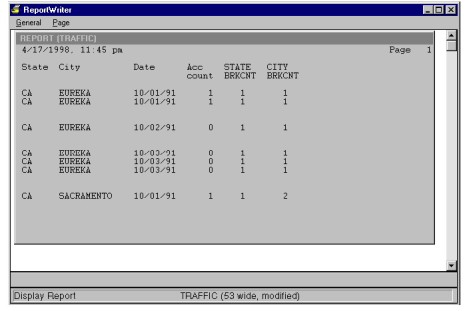%DATE

Returns the current date as an eight-digit value of the storage format YYYYMMDD. To use this function in a calculation field, select a type of Date and a length of 8, as shown in figure 3.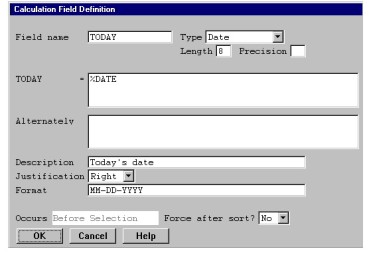%DATE is not explicitly supported in calculations on date fields. For example, you can’t subtract %DATE from another date. You must first assign %DATE into a calculation field whose type is Date. You can then use that calculation field in another calculation field that subtracts one field from another.

%TIME

Returns the current time as a four-digit value of the storage format HHMM. To use this function in a calculation field, select a type of Time and a length of 4.

%RECNUM

Returns the current record number (sequence number) being read in the primary file.

You can use the %RECNUM intrinsic in a calculation field and then use that calculation field in a selection criterion to optimize relative (primary) files. See Understanding ReportWriter optimization for more information about optimizing files.

%SUM(array_field[beg:end])

Returns the sum of a one-dimensional, numeric array (decimal or implied-decimal), where array_field is the name of the arrayed field (with or without the file definition and/or structure name), beg is a decimal field or literal that specifies the first element to include in the sum, and end is a decimal field or literal that specifies the last element to include in the sum.

If beg is greater than end, the sum will be 0. If beg or end is less than 1 or greater than the number of elements in array_field, a nonfatal subscript error will be generated.

As an example, we’ve created a calculation field named WK_SALES that has the characteristics shown in figure 4.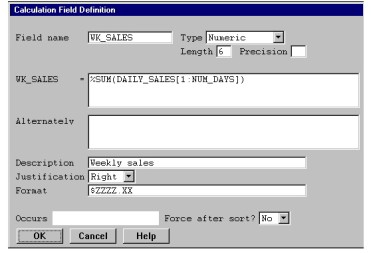%GOTREC(file)

You can use this function when you’re doing multiple projections (see Defining a multiple projection). %GOTREC returns a value of 1 if a record was read from the specified file or a value of 0 if no record was read.

You can specify file as the file definition name, the structure name, or a file definition/structure name combination, as long as file is unique to the set of file/structure combinations used in the report.

Once this value has been stored in the calculation field, you can use it as a conditional for selections, printing, or additional calculations.

## Assigning a conditional to a calculation field

You can define and assign a conditional to your temporary calculation field from the Calculation Field Definition window.

 1 Select Field functions > Specify conditions.

The Condition Criteria list is displayed. If you haven’t defined any conditions for the calculation field, this window is empty and the Criterion Definition window overlays it. As you define conditions, they are added to the Condition Criteria list.

 2 See Assigning a conditional to a field for detailed instructions on specifying a conditional.

Once you’ve defined the first condition, you can add additional conditions to this list by selecting Condition functions > Add condition. You can connect five conditions together (with AND and OR) and assign them to a calculation field.

To copy, edit, move, or delete a conditional, refer to the following:

 3 To return to the Calculation Field Definition window, press the Exit shortcut from the Condition Criteria window.

When a conditional is assigned to a calculation field, the word “Conditioned” is displayed below the Alternately field.